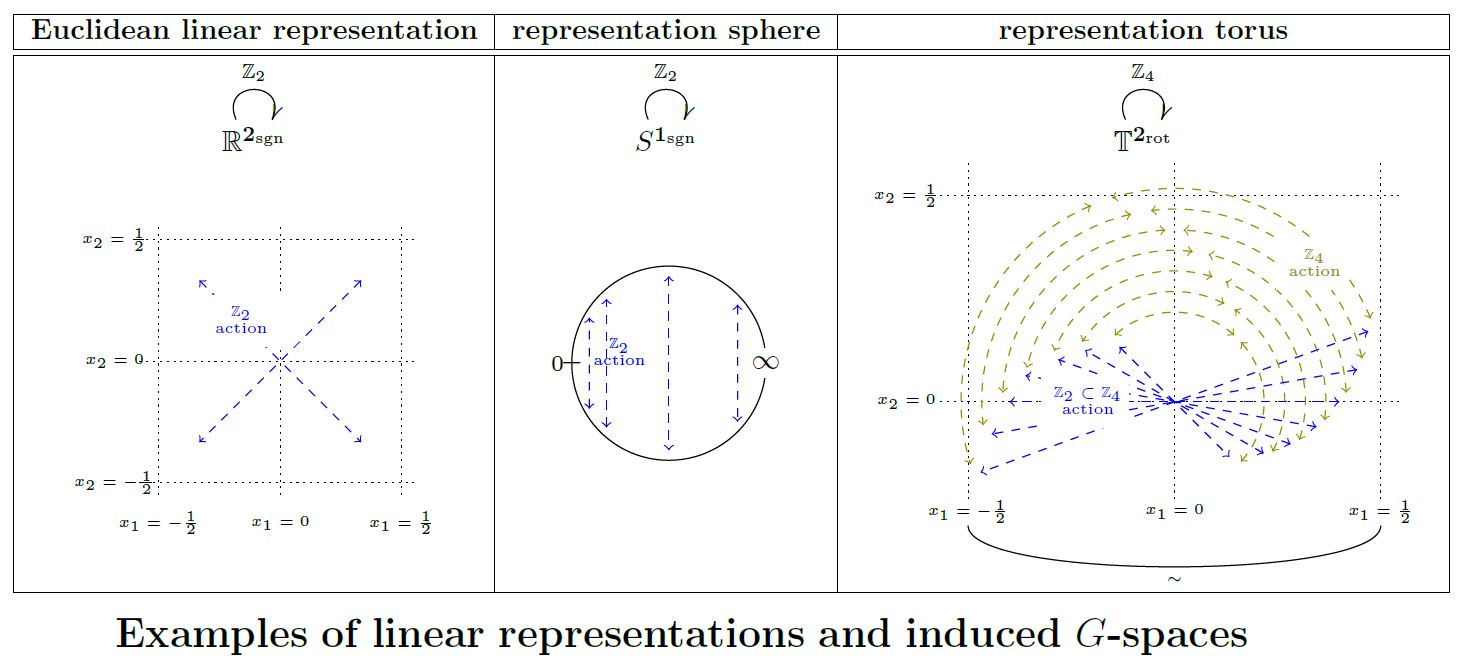# nLab topological G-space

Contents

### Context

#### Representation theory

representation theory

geometric representation theory

# Contents

## Idea

In the context of topology, a topological $G$-space (traditionally just $G$-space, for short, if the context is clear) is a topological space equipped with an action of a topological group $G$ (often, but crucially not always, taken to be a finite group).

The canonical homomorphisms of topological $G$-spaces are $G$-equivariant continuous functions, and the canonical choice of homotopies between these are $G$-equivariant continuous homotopies (for trivial $G$-action on the interval). A $G$-equivariant version of the Whitehead theorem says that on G-CW complexes these $G$-equivariant homotopy equivalences are equivalently those maps that induce weak homotopy equivalences on all fixed point spaces for all subgroups of $G$ (compact subgroups, if $G$ is allowed to be a Lie group).

By Elmendorf's theorem, this, in turn, is equivalent to the (∞,1)-presheaves over the orbit category of $G$. See below at In topological spaces – Homotopy theory.

See (Henriques-Gepner 07) for expression in terms of topological groupoids/orbispaces.

In the context of stable homotopy theory the stabilization of $G$-spaces is given by spectra with G-action; these lead to equivariant stable homotopy theory. See there for more details. (But beware that in this context one considers the richer concept of G-spectra, which have a forgetful functor to spectra with G-action but better homotopy theoretic properties. ) The union of this as $G$ is allowed to vary is the global equivariant stable homotopy theory.

## Properties

### Model structure and homotopy theory

The standard homotopy theory on $G$-spaces used in equivariant homotopy theory considers weak equivalences which are weak homotopy equivalence on all (ordinary) fixed point spaces for all suitable subgroups. By Elmendorf's theorem, this is equivalent to (∞,1)-presheaves over the orbit category of $G$.

On the other hand there is also the standard homotopy theory of infinity-actions, presented by the Borel model structure, in this context also called the “coarse” or “naive” equivariant model structure (Guillou).

## Examples

We discuss some classes of examples of G-spaces.

### Euclidean $G$-spaces

Let $V \in RO(G)$ be an orthogonal linear representation of a finite group $G$ on a real vector space $V$. Then the underlying Euclidean space $\mathbb{R}^V$ inherits the structure of a G-space

We may call this the Euclidean G-space associated with the linear representation $V$.

### Representation spheres

Let $V \in RO(G)$ be an orthogonal linear representation of a finite group $G$ on a real vector space $V$. Then the one-point compactification of the underlying Euclidean space $\mathbb{R}^V$ inherits the structure of a G-space with the point at infinity a fixed point. This is called the $V$-representation sphere

### Representation tori

Let $V \in RO(G)$ be an orthogonal linear representation of a finite group $G$ on a real vector space $V$.

If $G$ is the point group of a crystallographic group inside the Euclidean group

$N \rtimes G \hookrightarrow Iso(\mathbb{R}^V)$

then the $G$-action on the Euclidean space $\mathbb{R}^V$ descends to the quotient by the action of the translational normal subgroup lattice $N$ (this Prop.). The resulting $G$-space is an n-torus with $G$-action, which might be called the representation torus of $V$graphics grabbed from SS 19

### G-CW complexes

See at G-CW complex.

Rezk-global equivariant homotopy theory:

cohesive (∞,1)-toposits (∞,1)-sitebase (∞,1)-toposits (∞,1)-site
global equivariant homotopy theory $PSh_\infty(Glo)$global equivariant indexing category $Glo$∞Grpd $\simeq PSh_\infty(\ast)$point
sliced over terminal orbispace: $PSh_\infty(Glo)_{/\mathcal{N}}$$Glo_{/\mathcal{N}}$orbispaces $PSh_\infty(Orb)$global orbit category
sliced over $\mathbf{B}G$: $PSh_\infty(Glo)_{/\mathbf{B}G}$$Glo_{/\mathbf{B}G}$$G$-equivariant homotopy theory of G-spaces $L_{we} G Top \simeq PSh_\infty(Orb_G)$$G$-orbit category $Orb_{/\mathbf{B}G} = Orb_G$